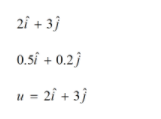Mechanics homework Help at TutorEye

## Mechanics :

Mechanics is the study of motion of objects and its causes in relation with the force and displacement.  It also deals with the subsequent effects of forces on the bodies and its environment. Classical mechanics relates motion of objects with the application of various external forces and quantum mechanics describes the phenomena of motion at atomic level.

## Mechanics Sample Questions:

Question 1: Which equation of Impulse is derived from Newton's third law of motion?

a) F.dt = m.da          b) F.dt = m.dv

c) F.dt = ma.dv        d) F.dt = mv.dv

Explanation:  According to Newton’s second law,  Momentum P = mv

Question 2: Given equation shows the relation of velocity w.r.t. to displacement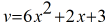. Find the acceleration at 2m.

a)   806           b) 622

c)   702           d) 812

Explanation: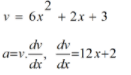Question 3: For a body to be in static equilibrium, which of the following conditions should be satisfied?

(a)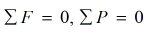(b)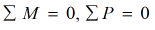(c)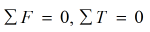(d)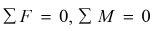Answer: (d)Explanation: For a body to be in static equilibrium, the sum of all the

Question 4:  Area moment of inertia is defined in which of the following deformation of solid bodies.

a) Bending, Twisting

b) Twisting, Rotating

c) Rolling, Bending

d) Bending, Rolling

Explanation: Area moment of inertia of any body's resistance to rotational deformation.

Question 5: What is the expression of torque for a body travelling in circular motion with angular acceleration ???? and moment of inertia I.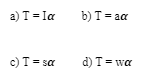Answer: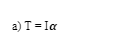Explanation: According to Newton’s second law of motion, F = ma

Question 6: In a projectile motion, the ratio of maximum height reached to the time of flight is

a)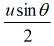b)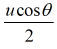c)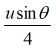d)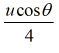Answer:  c)Explanation: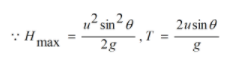Question 7: The ratio of mass moment of inertia of hollow sphere to the solid sphere is

a) 2/3      b) 5/3

c) 3/5      d) 3/2

Explanation: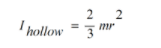Question 8: In equilibrium, forces acting on the body is

a) concurrent

b) concurrent, non-coplanar

c) coplanar, non-concurrent

d) concurrent, coplanar

Explanation: For a body to be in static equilibrium, the forces

Question 9: A horizontal force of 5 N is required to hold the box stationary against a wall. The coefficient of static friction between block and wall is 0.5. Find the weight of the block.

a) 2.5 N      b) 1 N

c) 1.5 N      d) 2 N

Explanation:  For the box to be stationary, Friction force acting in the upward direction balances the weight of the body.

Question 10: A body has initial velocity and acceleration as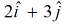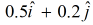respectively. Find the velocity after 5 seconds.

a) 7 m/s        b) 6 m/s

c)  2 m/s       d) 5 m/s

Explanation: